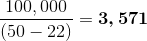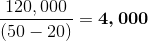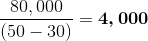# A firm is considering three capacity alternatives: A, B, and C. Alternative A would have an...

## Question:

A firm is considering three capacity alternatives: A, B, and C. Alternative A would have an annual fixed cost of $100,000 and variable costs of$22 per unit. Alternative B would have annual fixed costs of $120,000 and variable costs of$20 per unit. Alternative C would have fixed costs of $80,000 and variable costs of$30 per unit. Revenue is expected to be $50 per unit. a. Which alternative has the lowest break-even quantity? b. Which alternative will produce the highest profits for an annual output of 10,000 units? c. Which alternative would require the lowest volume of output to generate an annual profit of$50,000?

## Fixed Costs vs Variable costs

When doing a break-even analysis, it is useful to understand the difference between fixed and variable costs. A company's fixed costs are those that remain the same no matter how much or how little it sells. An example could be the monthly lease on a factory, which would be a constant amount regardless of the company's monthly sales. On the other hand, a variable cost is one that would change in line with increases or decreases in sales. A good example would be a manufacturing company's raw materials costs, which would naturally vary according to the level of the company's sales.

a. When variable costs are directly proportional to sales, the simple break-even formula can be used to calculate break-even quantity.
The formula is: {eq}Q = F/(p-v) {/eq}, where Q = break-even quantity, F = total fixed costs, p = price (or revenue) per unit, and v = variable costs per unit.

Thus, here are the break-even quantities for each of the 3 alternatives:

• Alternative A:• Alternative B:• Alternative C:ANSWER: The alternative with the lowest break-even quantity is A.

b. For the second question, use the formula: {eq}Profit = Q(p-v) - F {/eq}
Substitute 10,000 for Q and the same values for F, p, and v as in question a.

• Alternative A profits: 10,000(50-22) - 100,000 = $180,000 • Alternative B profits: 10,000(50-20) - 120,000 =$180,000
• Alternative C profits: 10,000(50-30) - 80,000 = $120,000 ANSWER: The alternative with the highest profits for annual output of 10,000 units is A or B. c. Use the same formula as in b. above, but substitute$50,000 for Profit and solve for Q. Thus, {eq}Q = (50,000+F)/(P-v) {/eq} Notice this is the same equation as question a., except that $50,000 has been added to the numerator. Here are the volumes (rounded to the nearest unit) needed to generate an annual profit of$50,000 :

• Alternative A: {eq}(50,000+100,000)/(50-22) = 150,000/28 = 5,357 {/eq}
• Alternative B: {eq}(50,000+120,000)/(50-20) = 170,000/30 = 5,667 {/eq}
• Alternative C: {eq}(50,000+80,000)/(50-30) = 130,000/20 = 6,500 {/eq}

ANSWER: The alternative with the lowest volume of output to generate a \$50,000 annual profit is A.